IV. TRANSFORMATIONS AND FUNCTIONS.

TRANSFORMATIONS.

In previous lessons, you were introduced to the simplest projector for shadow theater. It requires a light source (lamp, flashlight, LED, sun) and a screen (sheet of paper, wall, floor surface and other surfaces). You already know that if the rays of light are parallel to each other, then such a projection is called parallel, and if not, then perspective. The latter is so called because in the projection there will be a point, or a perspective, where the projections of parallel lines converge. Here's an interesting feature. In perspective projection, rays of light radiate from one point (Fig. 1).Fig. 1. The principle of operation of a projector with a point light source. Such a projector creates a perspective projection. In the case of perspective projections, the dimensions are changed. For example, points on the body transform to BD points on the screen, and segment AC is smaller than segment BD.

And in the projection in the Figure 2, the shadows of parallel lines (in the figure, parallel lines are indicated in red, and their shadows in black) will also tend to go through a common point (point K in the figure). However, they will never pass through it, but will only tend to reach it.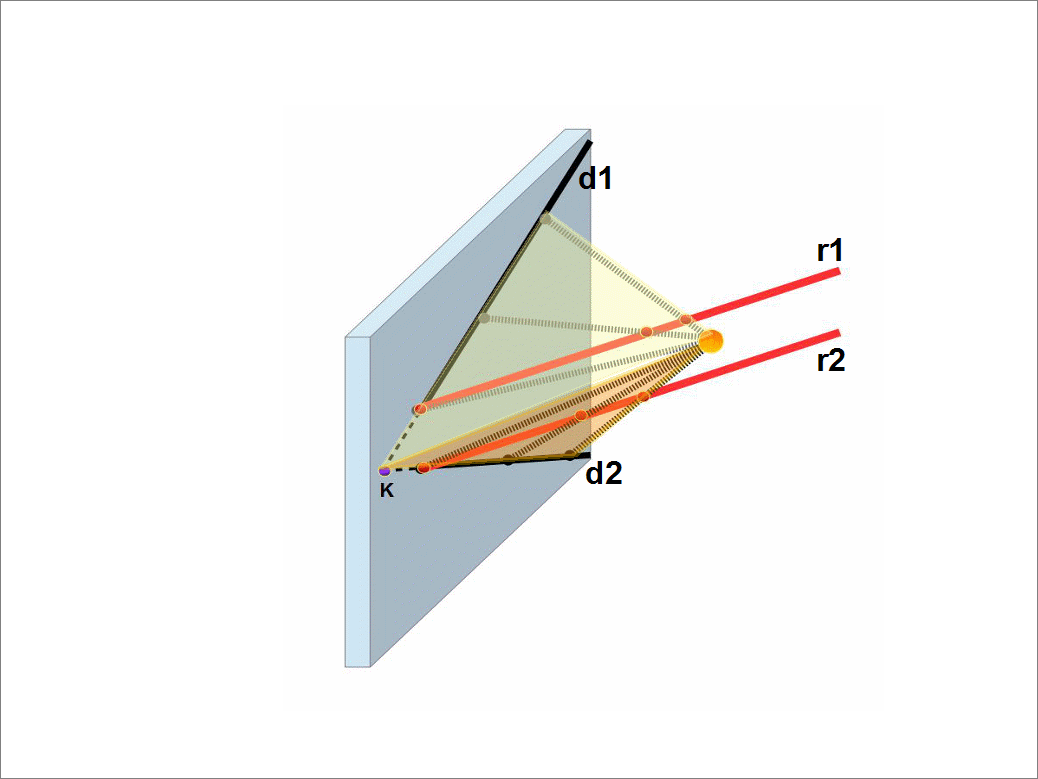Fig. 2. Perspective projection of parallel lines onto a plane. The light source is shown with an orange circle. Small dashed lines show several rays of light emitted from the source and passing through points on parallel straight lines r1 and r2 (shown in red). These points are projected onto the black points of the projection plane. Black points lie on black rays d1 and d2, into which red parallel lines are transformed as a result of projection. The resulting projections of red lines (or we can say shadows of red lines) intersect in the point K when extended.

It is interesting if we draw more straight lines that are parallel to the red lines, all their projections onto plane will converge at the same point K. This means that the point K will be the intersection point of the projections of a given family of parallel lines. If we take another family of parallel lines, then their projections onto the plane will have a different intersection point. For a family of straight lines parallel to the projection plane (that is, they do not intersect with the plane), the projections of parallel straight lines will also be parallel.

You can check this yourself by playing with the projections of parallel rails (wires, bars, strings) and your simplest projector. Think for yourself how best to do it. You might be familiar with perspective (or they are also called central) projections from pictures and photographs. Fig. Figure 3 gives an example of typical one- and two-perspective drawings.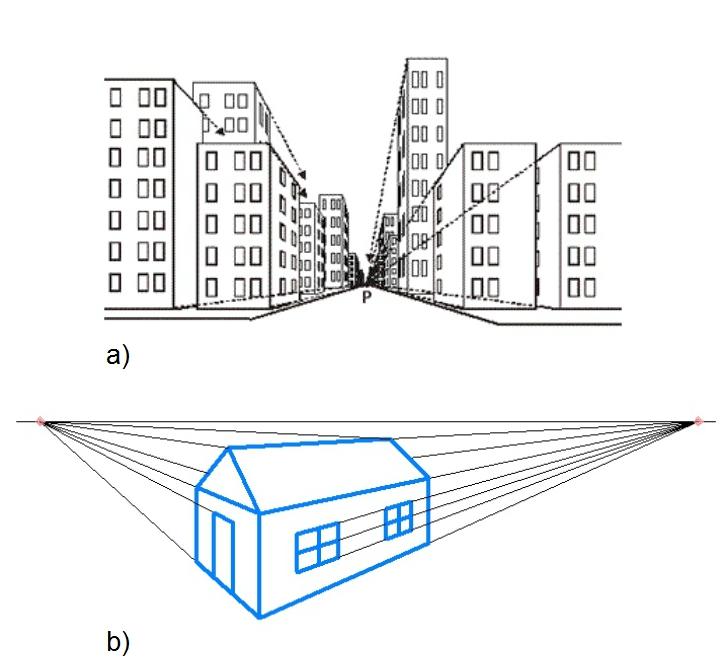Fig 3. a) Drawing with perspective (point P). As you can clearly see, all the parallel lines of roofs, sidewalks, floors converge at point R. At the same time, the lines of roofs, floors, sidewalks parallel to the plane of the drawing (parts of buildings and roads that are looking at us) remain parallel in the drawing itself, and have no intersection points.
b) Drawing with two perspectives. Two original families of parallel lines are visible here. Each of them has its own perspective point.
A good example of parallel projection is the children's toy shown in photo 4a. The principle of its operation can be understood from photo 4b.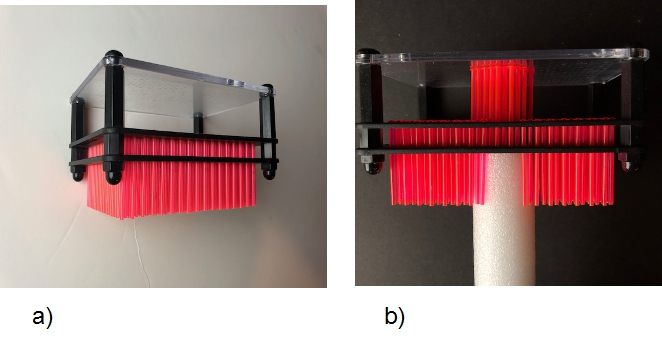Fig. 4. a) A pin art toy is the 3-dimensional transformer; b) the principle of operation of the pin art toy is based on the displacement of parallel red needles following relief changes.Fig. 5. The picture explains how the pin art toy is operating. If we will put an additional plane (for example a glass sheet) on top of the pin art, then we can see how points A and C of the body transform to points B and D on the plane. This transformation is an example of parallel projection.

In Fig. 5, the operating principle of the pin art toy is shown schematically. When the body rises, it pushes the needles, and they rise too. In this case, each point A of the body corresponds to its own point B on the surface of the needles. Thus, the surface of the body is, as it were, transformed into the surface of the needles. We can say that when the surface of the needles touches the glass, the surface of the body is projected at points on the glass surface.

With this projection, each point of the body is projected along the corresponding needle and the needles will be the projection lines. This will be a parallel projection since all projection lines are parallel. Moreover, it will be an orthogonal projection, since the projection lines (needles) are perpendicular to the glass surface on which we are projecting the body.

A feature of orthogonal drawings is the preservation of distances during projection and parallel lines are always converted to parallel lines on the other surface. For example, in Figure 5, the AC segment is projected into the BD segment. The lengths of the segments are equal. You probably already guessed where you've seen orthographic projections. Such an example was the projection of technical drawings with a scale of 1: 1. Figure 6 shows other examples of orthographic projections made with such a projector.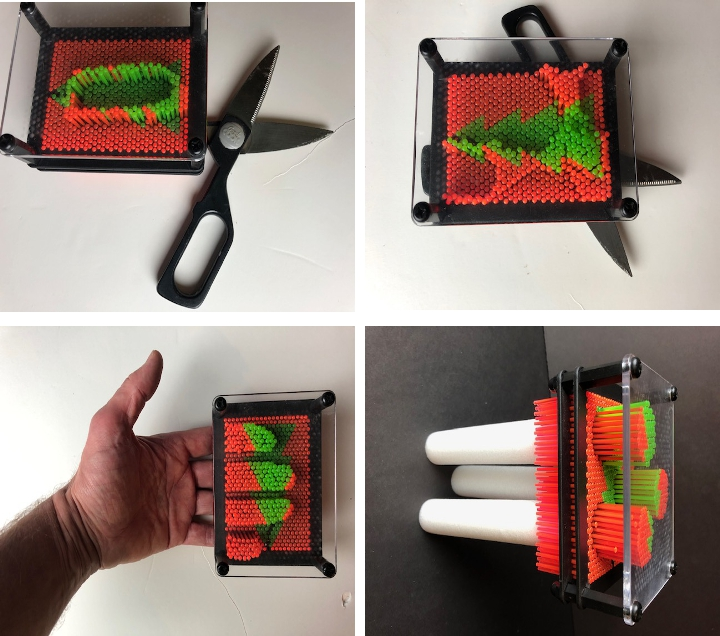Fig. 6. Examples of orthogonal projections made with a pin art toy.

A parallel projection preserves the parallelism of lines during projection, like an orthogonal projection, but it can change the sizes of lines and bodies. The differences between parallel and perspective projections are shown in Figure 7.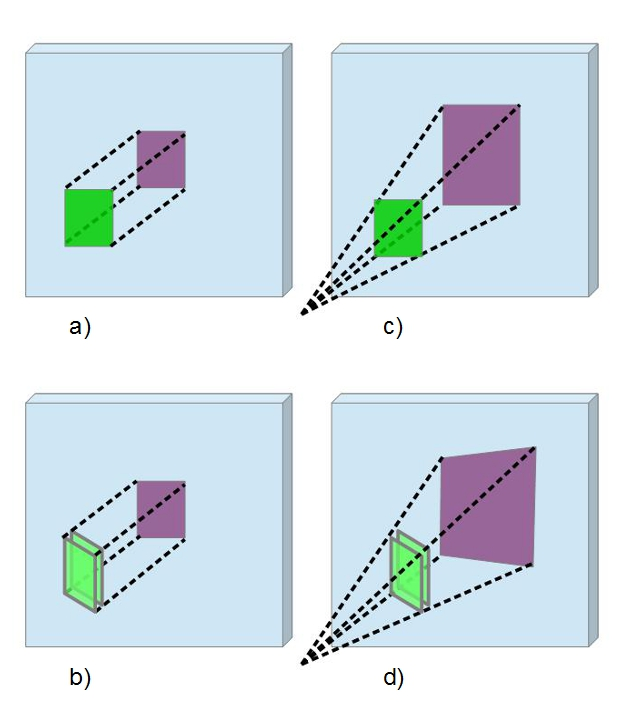Fig. 7. Left (images a) and b))- parallel projection; on the right , images c) and d) are a perspective projection. Projected shapes are shown in purple. The original rectangle is shown in green. In images a) and b), the projection plane is parallel to the object, and the rectangular shape is projected into a rectangle. In images b) and d), the projection plane is not parallel to the original figure and the rectangle is distorted. In the image b), the projected figure is a parallelogram, which at some angles of inclination of the projection plane can be a rectangle. In the image d), the projected figure is a quadrilateral, which can be a trapezoid for some special angles of the projection plane.

What parallel and perspective projections have in common is that
they transform straight lines into straight lines without bending them. These are linear transformations.

II. Basic mathematical concepts that we need to know now.
So physics is the study of natural phenomena. What do we call phenomena? By this term we mean transformations in nature, as a result of which the position in space and / or the characteristics of the object under study change. Therefore, in order to describe the phenomena taking place, it is very important for us to know what transformation is. You just met one example of transformations, projection. When projecting, one shape is transformed into another.
As the name suggests, transformation describes any phenomenon where something is transformed. In the language of mathematics, it sounds a little different.
First, the vague concept of "something" is replaced by a more specific "set of points". In mathematics, the word point can mean anything: numbers, balls, table cells, shadow points, running methods, rainbow colors, etc. Second, transformation means transforming that point to another point. By transforming all points of the original set to "others", we thus create a new set of transformed points (Fig. 8):Fig. 8. Transforming the original set of points to the transformed one.

Let's see what the transformation is for the example of roasting chicken (Fig. 9). The arrows show which points of the original carcass are converted to individual points of the roasted one. This conversion is the transformation of the original carcass points to the points of the roasted chicken. It is clear that many more could be taken as an example of such points. Mathematically, the entire chicken carcass is made up of infinitely small dots. If we begin to simulate the behavior of a carcass during roasting from a physical point of view, then each such point contains a very small volume of a chicken carcass. And the smaller this volume, the more such points.

In general, not only material points and their coordinates can be transformed. Different quantities characterizing technological processes or the state of the body can also be transformed. For example, in the case of chicken, this could be the temperature of the carcass at individual points at different times during the frying.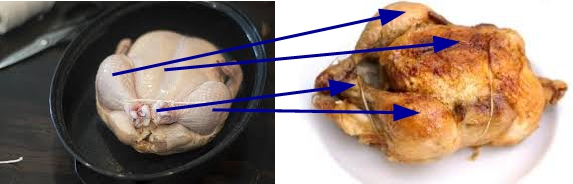Fig. 9. Transformations when roasting chicken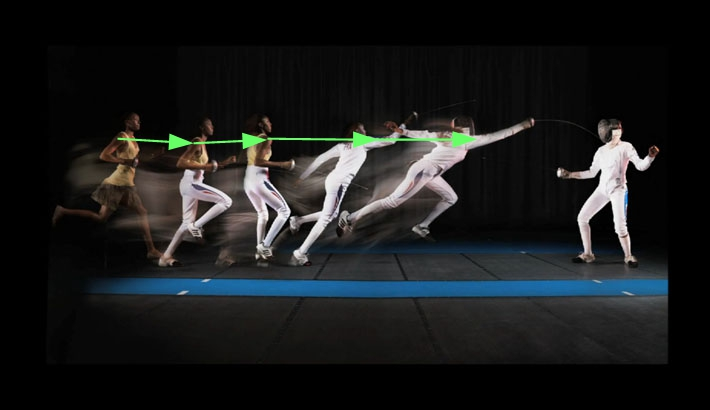Fig. 10. Athlete transformations performed using computer graphics.

The photograph in Fig. 10 illustrates the virtual transformations of a person carried out using computer graphics. The transformation of just one point of the image of a person is shown with green arrows. Even in the process of creating the simplest image on a computer, for example, what you see in front of you, each dot-pixel of the original photo is the point of the original real object that is transformed several times before you see it on the monitor screen.

These two photographs of a running person show the transformation of the position of the points of the runner's body in space. In the first picture, again, only a couple of points are taken as an example. You can define the transformations of the rest of the body points yourself. The second photo shows the transformation of the stripes on the runner's clothing in space during the run. Thus, the movement of body parts during running is studied. The knowledge of how their position relative to each other is transformed is then used in computer graphics when drawing cartoons and creating modern feature films.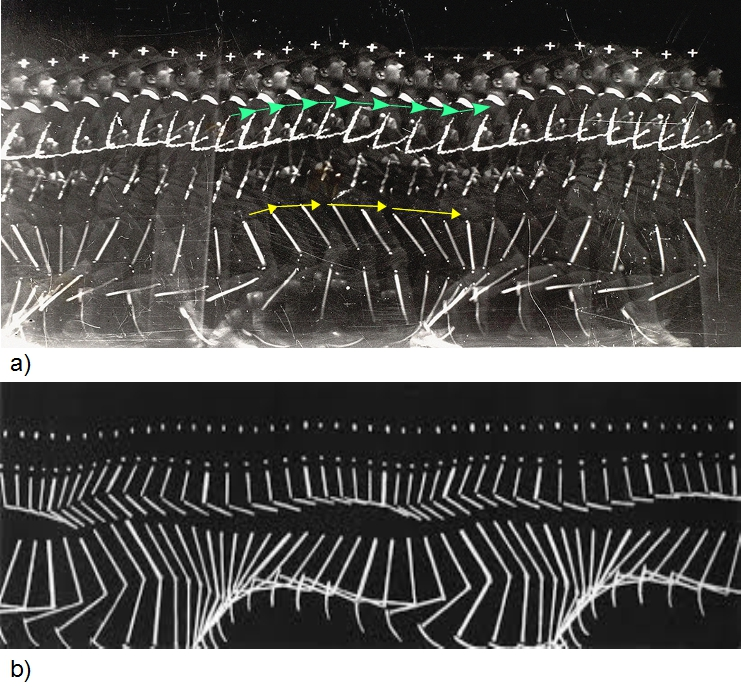Fig.11. Changes in the position of parts of the human body when running.

To simplify our notes, let's call the original set of points the letter X and the set of transformed points the letter Y. These can be any other letters and signs, but for now we'll use X and Y.

FUNCTIONS.

How exactly each point of the original set X is transformed into a point of the set Y is determined by the function that defines this transformation. In other words, the function describes the rules by which the transformation is performed. There are only 5 different ways of defining a function: using 1) tables, 2) graphics, 3) analytical expression (equation), 4) verbal description, and 5) recurrent way (when there are chain of repeated transformations, and each subsequent transformation is determined by the previous one).

Since the function describes transformations, it is always necessary to specify the initial set to define it. In function language, the set X is called domain; and the set Y is called codomain. The name codomain highlights that each point of the Y set has related original point of the X domain, or each point of Y set is a copoint of the related point of the X set. This can be briefly expressed in words: Y is a function of X, which is usually written in the language of mathematics like this Y= f(X), or X-> Y. Another name of the Y set is a range. Usually we will use name “range” (Fig.12). Our optical projections are a practical case of mathematics transformations. Therefore the Y set sometimes is called as an image set.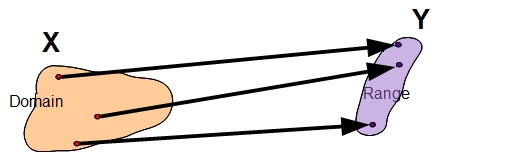Fig.12. The same image as in Fig.8 shows which set is а domain and which is а range.

Let's practice a little in speaking mathematical language.
Example 1.
We have three points of domain X which have been converted into three points of the codamain Y like in Figure 8. In mathematical language, we can say that we have points x1, x2, and x3, and they were transformed into points y1, y2, and y3, respectively. The sentence, “domain X was transformed into three points of the codamain Y” can be written as Y=f(X). The sentence, “points x1, x2, and x3, and they were converted into points y1, y2, and y3, respectively” can be written as y1=f(x1), y2=f(x2), and y3 = f(x3).
Should we take two more points in the domain X, we can write for these two additional points the following expressions: y4= f(x4) and y5= f(x5).
The phrase “ y1 is a copoint of the x1” means that each function creates related to each other pair of points x1 and y1 and it can be written as (x1,y1).
Similar denotes can be used for any other pair of points connected each to other by the function. For example, for points x4 and y4 we can write (x4, y4).
For us, transformation and function are very important concepts because we will be using them for programming and in OpenOffice spreadsheets. We will begin the study of functions by setting them with graphs and tables.
In previous lessons, you played naval combat on checkered paper and you know what coordinates are. Consider a checkered field similar to a naval battlefield, only to designate columns we will replace letters with numbers. That is, we write the numbers in the same way as for rows. In addition, we will increase the number of cells to 15. Then our horizontal and vertical coordinates of the cells will be integers from 1 to 15. The horizontal coordinate will be called the X-axis, and the vertical Y-axis.
Coordinates, as before, will be a pair of numbers, which we will write in brackets like this: (X, Y), where instead of X and Y we will write down the corresponding values ​​of the coordinates of the cells. Please note, since we are not using letters for coordinates now, so that there is no confusion, the first digit will always be the X coordinate, and the second - the Y coordinate. As you see, this denote is similar to the denote of the paired points for functions. Let's add a point with coordinates (0,0). We will get the following field (Fig. 13):Fig. 13. Cartesian coordinates field

Now, we draw three different lines on this field (Fig. 14):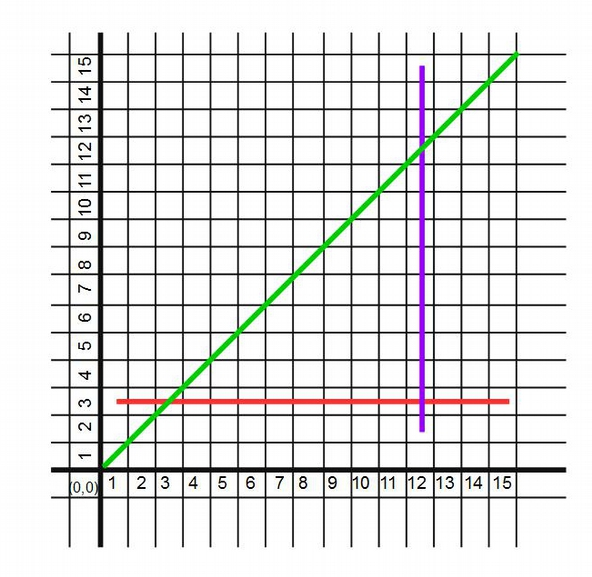Fig. 14. Three lines on the coordinate plane. Each of the lines defines its own function, which determines the X coordinate and the Y coordinate for each point of the line.

Pay attention, by drawing a line, we set a rule according to which each point of the X coordinate can be associated with a certain point of the Y coordinate (in this case, each point equals to cell, so we have a large points). For example, a point along the X axis has a coordinate of 7. Then if we take a green line, then the point of the line with the X coordinate is 7 has a Y coordinate equal to 7. And the red line, in a similar way, will match point X equal to 7 point Y equal to 3. This means that knowing the line, we will always can find the corresponding point or copoint of the range Y for each point of the domain X. And each line determines a certain function, which transformed points of the X domain into points of the Y range

Let's denote the function that determines the green line with letter g, red line with letter a, and violet line as v. Then green line function can be written as Y=g(X), the red line function as Y=r(X), and violet line function as Y=v(X). Then in mathematical language for the first point of the green line with coordinate 7, we can write 7=g(7), or we can write this as a pair of numbers: (7,7), or we can write x1=7 and y1=7. Similar for the red line, we can write 3=r(7), or (3,7), or x1=7 and y1=3.

Thus, any line on the coordinate plane defines a function that assigns each point on the X axis to a specific point on the Y axis. This means that any line specifies a rule for transforming points of the set X into points of the set Y. But of course, this rule works only for those points of X for which the line is defined. For example, the green and red lines are defined for points X from 1 to 15, but not defined for point X = 0. And the purple line is defined only for the point X = 12. The points at which the line is defined are the domain of the function, which is set by the line.

Now let's speak a little about computers. All modern computers use functions to operate and to perform different tasks. But a computer brain, the microprocessor, can work with numbers only. Even the most advanced modern computers cannot see as a human and cannot understand as a human what a line or any graphic represents. It does not speak as a human and cannot understand verbal descriptions as a human. It needs translators which will translate the graphic objects and verbal descriptions in the language of digits.

Now we will learn how to convert the graphical representation of the line to numerical representation, or in other words how to digitize the lines. So far we specified only one point per line. But one point is not enough to determine how this line differs from others or how this function differs from others functions. No one can say how looks the whole line if he can see only one point of the line. We should take more points and assign each of them the related (x,y) pair of numbers.

Now, let's imagine that we are playing in a sea battle and we need to accurately hit the lines. We will shoot at the points of the lines by setting their coordinates in the same way as we were shooting at the ships. We will record our exact shots in a table with two columns. In the first column, let's call it column X, we will write the x coordinate of points. In the second, in the Y column, we will write down the corresponding y coordinate for each point in the X column. And let us only have 9 shells to shoot. Then, for the green line, we can get the following table: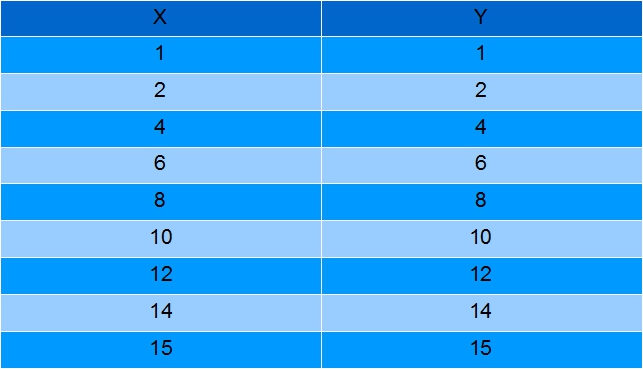This table defines a function whose domain and range contain 9 points each. This equals to a number of points of the line, which we digitized. For the first point, we have x1=1, y1=1; for the second point: x2=2, y2=2; for the third point: x3=4, y3=4; and so on until the ninth point with x9=15, y9=15.

Of course, you noticed that I did not digitize all points, but only the extreme ones and with even X. It was possible to digitize all points, and it was possible to digitize other points, for example, only with an odd X. The number of points is determined only by how accurately you want set your function. The minimum number of points that must be set for a straight line is 2 (why ??). The maximum number is equal to infinity, because unlike naval combat, there might be also fractional coordinates, for example, x value can be 3 and a half. And there are infinitely many fractions between integers. But it is always advisable to set extreme points, since they define the domain and range boundaries.
For the violet line, the table might look like this: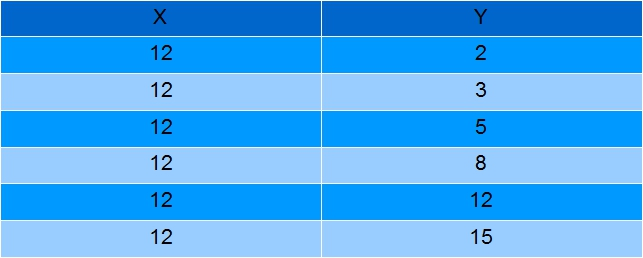In this case we have only 6 points of line which were digitized. According to the table, x1=12, y1=2 for the first point; x2=12, y2=3 for the second point; x3=12, y3=5 for the third point; x4=12, y4=8 for the fourth point; x5=12, y5=12 for the fifth point;and for the sixth point x6=12, y6=15. As you can see from the line, the line's function has domain that contains only one point, but range that contains 14 points at least if we count only integer numbers. Therefore all six numbers of the X domain in the table are equal: x1=x2=x3=x4=x5=x6=12.

Introduction to programming.

First, you should specify your operating system and download OpenOffice (click on Download full installation). Then the program will download the installation file to your computer. It will take some time. Then start the executive program by clicking on the saved file. Then follow the installation instructions. Please be patient: the installation of the program can take up to half an hour. After installing OpenOffice, you need to open OpenOffice.org Calc.
Here are tutorials that will explain how to use spreadsheets in OpenOffice Spreadsheets (all videos in Russian. You can find the related videos in English on youtube youself).

Introduction. Using tables as a calculator: https://youtu.be/GPvK7brpOzo
Introduction. Functions and graphs:
https://youtu.be/HgsfXzyRwSg
Working with charts. We make the multiplication table:
https://youtu.be/2vymTy2FR2k
https://youtu.be/PA57siQBxDM
https://youtu.be/c48ivzUgqYI
The basic information is in the tutorial videos, but just in case, here's a text guide on how to get started with spreadsheets in OpenOffice Spreadsheets.

After the corresponding program is opened, you will see a table (Fig. 1a). The table itself resembles the "Sea Battle" game field. As in naval combat, each cell of the table has a coordinate, for example A1 or C5. But the table field is certainly much more interesting. The reason for this is that it is inherently very close to the computer's memory, which also has two-dimensional coordinates of its memory cells. As well as in the computer memory, various information can be stored in the table cells. It can be viewed by hovering the cursor over the corresponding cell and clicking the left mouse button. The cell’s contents will appear in the field “Input Line”outlined with a purple curve in the Figure 1.a. And vice versa, if we select a cell and write something in this field, and then press Enter, then what was written will be saved in the previously selected cell.

What can be stored in a table cell? Numbers, text and functions. The table itself distinguishes decimal numbers from text and from functions. Distinguishes according to a simple principle: what consists of numbers is a number. Anything that consists of letters or any characters, but the first character '(for example, abc or' 123) - refers to string, i.e. text information. If the first is the equal sign =, then this is a table function (for example, = sum (A1: D1) means a function that sums up the numbers in cells from A1 to D1: A1, B1, C1, and D1).

As well as in computer memory, two types of addressing are practically implemented in tables: absolute and relative. With absolute addressing, we find or store data in the cell with the specified address. For example, we can save the number 7 in cell A1. To do this, move the mouse cursor over cell A1 and type the number 7. Then press the Enter key to save the number in the cell. If now again hover the cursor over the cell with the number 7, then in the Input Line field you will see the same “7” (the value field is circled in a purple ellipse in Fig.1.a.). Use the same Input Line to edit / correct the contents of the cell.

With relative addressing, we find or store in the cell the address of another cell that contains the data needed. For example, if we will save in the cell B3, the next expression
“=A1”,
or “=\$A\$1” ,
or “=A\$1”,
or “=\$A1”,
and push the Enter key, then we see number 7 in the cell B1 (Fig.15). However, if we will navigate cursor over the cell B3 and click with left button, then we see the expression “=A1” in the Input Line. Later you will learn why we need these both two address schemes, and effect of using the sign of dollar.

The sequence of actions when editing the cell is usual. First, hold down the left mouse button and select by dragging the mouse's cursor over text that you want to fix. Then you print the corrections and push the Enter key. The same effect can be achieved by selecting with the arrows and simultaneously pressing the Shift key on the keyboard.Fig. 15. a) View of an open sheet OpenOffice table. Note the tables contain 3 sheets. The first one is open (circled in orange). The name of Sheet1 can be edited by double-clicking on it and entering the desired name in the window that appears; b) writing and reading data in table cells. In both cases, you first need to move the cursor over the required cell of the table. Then click with the left mouse button. This will select the cell and a thicker black frame will appear around it. For example, cell B3 is highlighted in the figure. In this case, the value of the cell is 7, and in the Input Line ​​it can be seen that the function = A1 is written in the cell, which equates its value to the value of cell A1. The contents of the cell can be changed. To do this, you can either simply print its new content, which will automatically be entered into the selected cell; or move the cursor over the Input Line ​​and click the left mouse button, and then make the necessary corrections. If at this moment you change your mind about correcting, then you can press the Esc button on the keyboard. This will deactivate the cell. After making corrections, press the Enter button to save the corrections in the cell.

Below is a list of other commonly used table functions that you don't know yet, but which we will be getting to know. Remember, if you would like to use any functions you should print sign “= “ first and then function:

= power (a; b) - means the expression ab, for example = power (2; 3) will give 23 = 8; = power (8; 1/3) will give 2. Note that Microsoft Excel spreadsheets separate numbers with just a comma instead of a semicolon. For example, in Excel you should write: = power (2,3). The values ​​of a and b can be specified using relative addressing. For example: = power (E3; F1) will raise the number at address E3 to the power of the number at address F1.

= sqrt (a) - means the square root of the number a. For example = sqrt (4) will give 2. As usual, you can use relative addressing.
To calculate the product of the values ​​stored in the cells, it is necessary to write down the product of the cell addresses. For example, writing = A2 * D3 will count the product of the data in cells A2 and D3. Similarly, you can find the result of division, addition or subtraction: = A2 / D3; = A2 + D3; = A2-D3. If you have numbers that just need to be added, for example, you need to add 23 and 45, then this can be done by writing = 23 + 45. But it is more useful to first save the data in cells, and then operate the cells using relative addressing.
= max (a: b) - finds the maximum value in cells with addresses from a to b. For example, = max (C3: C5) finds the maximum value in cells C3 through C5: C3, C4, C5.
Finding the minimum works in a similar way. For example, = min (C3: C5) will find the minimum value at the same addresses.

= pi () - will return the value of a number. Note the parentheses: they are empty.
= sin (b) – gives you Y coordinate of the end of the 1 unit long segment when another end of the segment in the origin of the coordinates and angle between the segment and axis X is equal to b (Fig.16).
= cos (b)- gives you X coordinate of the end of the 1 unit long segment when another end of the segment in the origin of the coordinates and angle between the segment and axis X is equal to b (Fig.16).

= DEGREES (b)- converts b radians to angle degreesFig. 16. cos(b) and sin(b) are x and y coordinates of the end of the 1 unit long segment, where b - is the angle between the segment and X axis.

= exp (a) - finds the value of the exponent of the number a, or if a is the address, then the exponent of the number at the address a. For example: = exp (2) is equal e*e, where e= 2.718, and this gives 7.39.

The following videos show you how to use OpenOffice Spreadsheets: